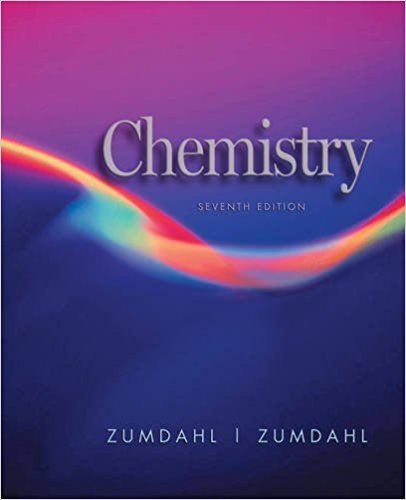×
×

# Solutions for Chapter 19: The Representative Elements: Groups 1A Through 4A## Full solutions for Chemistry | 7th Edition

ISBN: 9780618528448Solutions for Chapter 19: The Representative Elements: Groups 1A Through 4A

Solutions for Chapter 19
4 5 0 413 Reviews
16
5
##### ISBN: 9780618528448

This textbook survival guide was created for the textbook: Chemistry, edition: 7. Chapter 19: The Representative Elements: Groups 1A Through 4A includes 81 full step-by-step solutions. Since 81 problems in chapter 19: The Representative Elements: Groups 1A Through 4A have been answered, more than 51241 students have viewed full step-by-step solutions from this chapter. This expansive textbook survival guide covers the following chapters and their solutions. Chemistry was written by and is associated to the ISBN: 9780618528448.

Key Chemistry Terms and definitions covered in this textbook
• Anabolic steroid

A steroid hormone, such as testosterone, that promotes tissue and muscle growth and development

• Arene

A term used to classify benzene and its derivatives.

• back-side attack

In SN2 reactions, the side opposite the leaving group, which is where the nucleophile attacks.

• buffered solution (buffer)

A solution that undergoes a limited change in pH upon addition of a small amount of acid or base. (Section 17.2)

• chain-growth polymer

A polymer that is formed under conditions in which the monomers do not react directly with each other, but rather, each monomer is added to the growing chain, one at a time.

• doublet

In NMR spectroscopy, a signal that is comprised of two peaks.

• formula weight

The mass of the collection of atoms represented by a chemical formula. For example, the formula weight of NO2 (46.0 amu) is the sum of the masses of one nitrogen atom and two oxygen atoms. (Section 3.3)

• Fourier transform NMR (FT-NMR)

The modern NMR method that is based on a constant magnetic fi eld, a short pulse of electromagnetic radiation, and a mathematical Fourier transform to produce the spectrum

• Grignard reagent

A carbanion with the structure RMgX.

• intermetallic compound

A homogeneous alloy with definite properties and a fixed composition. Intermetallic compounds are stoichiometric compounds that form between metallic elements. (Section 12.3)

• isoelectric point (pI)

For an amino acid, the specific pH at which the concentration of the zwitterionic form reaches its maximum value.

• ketone

A compound in which the carbonyl group 1C “O2 occurs at the interior of a carbon chain and is therefore flanked by carbon atoms. (Section 24.4)

• kinetics

A term that refers to the rate of a reaction.

• molecule

A chemical combination of two or more atoms. (Sections 1.1 and 2.6)

• plane of symmetry

A plane that bisects a compound into two halves that are mirror images of each other.

• Racemic mixture

A mixture of equal amounts of two enantiomers.

• reactant

A starting substance in a chemical reaction; it appears to the left of the arrow in a chemical equation. (Section 3.1)

• semiconductor

A material that has electrical conductivity between that of a metal and that of an insulator. (Section 12.7)

• spin-spin splitting

A phenomenon observed most commonly for nonequivalent protons connected to adjacent carbon atoms, in which the multiplicity of each signal is affected by the other.

• stretching

In IR spectroscopy, atype of vibration that generally produces a signal in the diagnostic region of an IR spectrum.

×